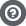Hello Guest

# glu functions LWJGL3

• 9 Replies
• 16491 Views####TheBoneJarmer

•• 70##### glu functions LWJGL3
« on: November 15, 2014, 19:23:55 »
Hey

I have been looking in the docs, but I am unable to find the glu functions like gluPerspective. What happend to them?

-TBJ####spasi

•• 2216##### Re: glu functions LWJGL3
« Reply #1 on: November 15, 2014, 19:54:54 »####TheBoneJarmer

•• 70##### Re: glu functions LWJGL3
« Reply #2 on: November 15, 2014, 20:34:40 »
I see, thanks! But will you leave it out for good or is there going to be a replacement?####kappa

•• 1316##### Re: glu functions LWJGL3
« Reply #3 on: November 15, 2014, 20:37:21 »
GLU is using pretty ancient OpenGL internally, almost all the projects using modern OpenGL don't use it anymore. Better to avoid it and use shaders together with a nice maths library.####TheBoneJarmer

•• 70##### Re: glu functions LWJGL3
« Reply #4 on: November 15, 2014, 20:48:11 »
I see. So, what can I use instead of gluPerspective then?####kappa

•• 1316##### Re: glu functions LWJGL3
« Reply #5 on: November 15, 2014, 20:55:02 »
Look up a few modern OpenGL tutorials, most will explain how it works.

In short its usually something along the lines that you multiple your vertices by a matrix before you send them to your shader for drawing (or multiply the vertices in your shader).

Such matrices are usually created using a maths library (or you write such code yourself). Matrices copying those provided by GLU would look something like this:

Code: [Select]
`import org.lwjgl.util.vector.Matrix4f;import org.lwjgl.util.vector.Vector3f;public class GLM { public static Matrix4f ortho(float left, float right, float bottom, float top, float zNear, float zFar) { Matrix4f m = new Matrix4f(); m.m00 = 2 / (right - left); m.m11 = 2 / (top - bottom); m.m22 = - 2 / (zFar - zNear); m.m30 = - (right + left) / (right - left); m.m31 = - (top + bottom) / (top - bottom); m.m32 = - (zFar + zNear) / (zFar - zNear); return m; } public static Matrix4f frustum(float left, float right,               float bottom, float top,               float zNear, float zFar) { Matrix4f m = new Matrix4f(); m.m00 = (2 * zNear) / (right - left); m.m11 = (2 * zNear) / (top - bottom); m.m20 = (right + left) / (right - left); m.m21 = (top + bottom) / (top - bottom); m.m22 = -(zFar + zNear) / (zFar - zNear); m.m23 = -1; m.m32 = -(2 * zFar * zNear) / (zFar - zNear); return m; } public static Matrix4f perspective(float fovy, float aspect, float zNear, float zFar) { float range = (float) Math.tan(Math.toRadians(fovy / 2)) * zNear; float left = -range * aspect; float right = range * aspect; float bottom = -range; float top = range; Matrix4f m = new Matrix4f(); m.m00 = (2 * zNear) / (right - left); m.m11 = (2 * zNear) / (top - bottom); m.m22 = - (zFar + zNear) / (zFar - zNear); m.m23 = - 1; m.m32 = - (2 * zFar * zNear) / (zFar - zNear); return m; } public static Matrix4f lookAt(Vector3f eye, Vector3f center, Vector3f up) { Vector3f forward = Vector3f.sub(center, eye, null); forward.normalise(forward); Vector3f u = up.normalise(null); Vector3f side = Vector3f.cross(forward, u, null); side.normalise(side); Vector3f.cross(side, forward, u); Matrix4f m = new Matrix4f(); m.m00 = side.x;        m.m10 = side.y;        m.m20 = side.z;                m.m01 = u.x;        m.m11 = u.y;        m.m21 = u.z;                m.m02 = -forward.x;        m.m12 = -forward.y;        m.m22 = -forward.z;         m.translate(new Vector3f(-eye.x, -eye.y, -eye.z));        return m; }}`####TheBoneJarmer

•• 70##### Re: glu functions LWJGL3
« Reply #6 on: November 15, 2014, 22:31:05 »
I see. And what about glFrustum?####kappa

•• 1316##### Re: glu functions LWJGL3
« Reply #7 on: November 15, 2014, 22:57:20 »
I see. And what about glFrustum?
same applies, its also deprecated in modern opengl. Matrix implementation of the same in the code above.####aabbcc1241

•• 2##### Re: glu functions LWJGL3
« Reply #8 on: January 07, 2015, 22:14:09 »
How about the gluSphere?
What is the LWJGL 3 replacement for rendering a sphere?####kappa

•• 1316##### Re: glu functions LWJGL3
« Reply #9 on: January 07, 2015, 22:27:07 »
There are plenty of tutorials and examples on how to render a sphere in OpenGL.

In any event as people keep asking about GLU (even though its not recommended), you can still use GLU with LWJGL3, its been ported to LWJGL3 in the LWJGLX project, just check the code out and use the org.lwjglx.util.glu.* package (yes it has a Sphere class in there too).